Deepak Scored 45->99%ile with Bounce Back Crack Course. You can do it too!

# What is P-N junction - Definition, Explanation, Types - eSaral

P-N junctions are formed by diffusing trivalent impurity to one-half side and pentavalent impurity to another side. If you want to know what is P-N junction then keep reading this article.

## P-N Junction

• By merely Joining the two pieces a P-N Junction cannot be formed.
• P-N junctions are formed by diffusing trivalent impurity to one-half side and pentavalent impurity to another side.
• The plane dividing the two zones is known as a junction.
• P-N junction is unohmic
• As P-type semiconductor has a high concentration of holes and N-type semiconductor has a high concentration of free electrons. there is a tendency of holes to diffuse over to the N side and electron to the P side.
• When the hole diffuses from the P to N side then this will neutralize with free-electron similarly when electron diffuse from the N to P side it will neutralize with the hole. So, a depletion layer is formed near the Jn.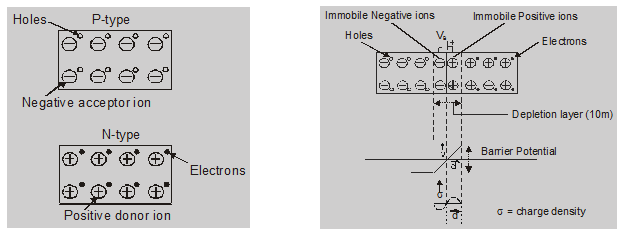### Depletion layer:

There is a barrier near a junction that opposes the flow of charge carrier is known as depletion layer width of the depletion layer is in micrometer order.

### Potential Barrier:

Potential developed in depletion layer is called P.B.

P-side is at lower potential and N-side is at higher potential.

P.B. for Ge  0.3 volt

P.B. for Si  0.7 volt

Electric field:

Electric field due to P.B.

$E=\frac{V}{d}$

For Ge

$E=\frac{0.3}{10^{-6}}$

$=3 \times 10^{5} \mathrm{~V} / \mathrm{m}$

order

$\mathrm{E} \approx 10^{5} \mathrm{~V} / \mathrm{m}$

the direction of E due to P.B. N to P-side

## P-N Junction with forward bias :

When the Positive terminal of a battery is connected to the P side and the negative terminal to the N side. Then PN Junction is in forwarding Bias

Forward bias reduces the potential barrier. More charge carriers diffuse across the junction.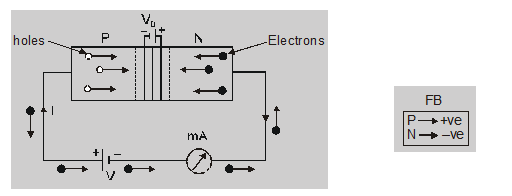Special Point:
• Potential barrier reduces
• Width of the depletion layer decreases
• P-N junction offers low resistance in forwarding bias.
• Forward current flow in a circuit
• The forward characteristic curve is shown in the figure.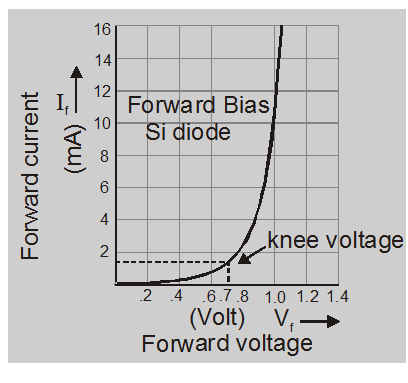• Forward dynamic resistance $r_{f}=\frac{\Delta V_{f}}{\Delta I_{f}} \cong 100 \Omega$
• Knee or cut in voltageGe  0.3 VSi  0.7 V
• Dependence of forward current on bias voltage $\mathrm{I}=\mathrm{I}_{0}\left[\mathrm{e}^{\frac{\mathrm{qV}}{\mathrm{kT}}}-1\right]$$\mathrm{e}^{\frac{\mathrm{qV}}{\mathrm{kT}}}>>1 I \approx I_{0} e^{\frac{+q V}{k T}} (Approximate exponential growth) I = Forward current \mathrm{I}_{0} = reverse saturation current k = Boltzman constant q = charge of electron V = forward voltage T = temperature ## P-N junction with reverse bias: When the positive terminal of a battery is connected to the N-side and the negative terminal is connected to the P-side. Holes in the P-region are attracted towards the negative terminal and the electrons in the N-region are attracted towards the positive terminal.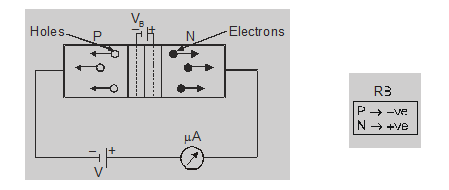Special Point: • The depletion layer increases for reverse biased. • Potential barrier increases • The reverse characteristic curve is shown in figure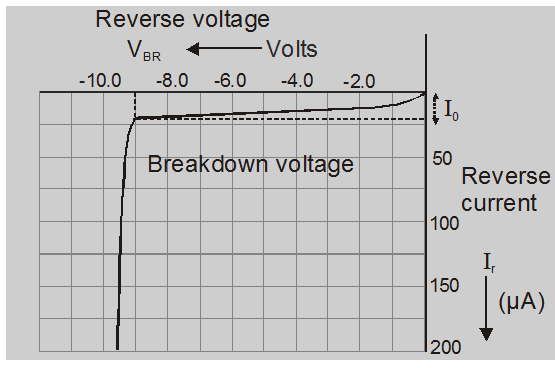• Very little current called reverse saturation current flows due to minority carrier flow.For Silicon = 10^{-9} AFor Germanium = 10^{-6} A • In reverse biased condition, junction behaves as a capacitor of few picofarads. • In reverse biased condition, junction behaves like high resistive material between two regions. • In reverse biased P-N diode behaves like an insulator. • Reverse resistance \mathrm{R}_{\mathrm{B}}=\frac{\Delta \mathrm{V}_{\mathrm{B}}}{\Delta \mathrm{I}_{\mathrm{B}}} \cong 10^{6} \Omega$$\frac{R_{B}}{R_{f}}=10^{3}$ : 1 for Geand

$\frac{R_{B}}{R_{f}}=10^{4}$ : 1 for Si
• Dependence of reverse current on bias volt. $\mathrm{I}_{\mathrm{r}}=\mathrm{I}_{0}\left[\mathrm{e}^{-\frac{\mathrm{q} \mathrm{V}}{\mathrm{kT}}}-1\right]$$\mathrm{e}^{-\frac{\mathrm{qV}}{\mathrm{kT}}}<<1$$\mathrm{I} \cong-\mathrm{I}_{0}$

Here $\mathrm{I}_{\mathrm{r}}$ = reverse current

$\mathrm{I}_{0}$ = reverse saturation current

V = applied voltage

q = charge of electron

T = temperature in kelvin
Special Point
• The diffusion current in the p-n junction is greater than the drift current in magnitude if the junction is forward biased.
• A hole diffuses from the p-side to the n-side in a p-n junction. This means that a bond is broken on the n-side and the electron freed from the bond jumps to a broken bond on the p-side to complete it.